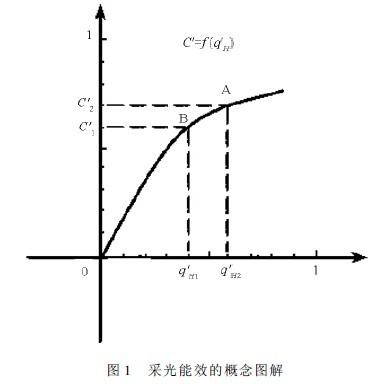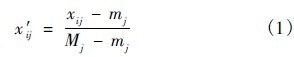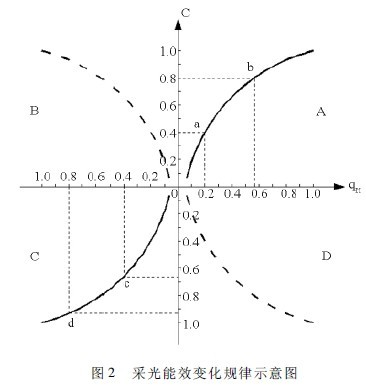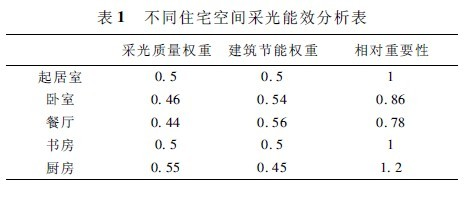1 Introduction

Louis Kang mentioned in a discussion of the window: "Window is a natural lighting device because it distributes light, which is different from ordinary artificial facilities. Although it is a device, a small machine, No engine is needed. It is just a carefully thought-out shape.â€ In the natural light environment design, the form of building, room and window determines the natural light intake, which can not only distribute natural light, but also influence Artificial lighting can affect heat intake or heat loss and ventilation. The windows provide the ideal lighting conditions for the interior, and the outdoor view is also unobstructed. People want to have this pleasant feeling, so large-area glass windows are becoming more and more common in houses, even in cold and cold regions. Windows are the weak link in energy-saving design. The thermal performance of windows is much worse than that of the outer wall. The larger the window, the more heat transfer the temperature difference is. Although the current form and glass technology can effectively reduce the heat transfer coefficient of the window, it is reduced. From the perspective of building energy consumption, China's energy-saving standards as a compulsory provision clearly limit the window-to-wall ratio of different residential buildings. In this case, the dual requirements of lighting and energy saving for the form of windows have formed a contradiction to some extent. For architects, how to effectively balance this contradiction in the early stage of architectural design through the design of buildings and windows. In response to this problem, this paper proposes the concept of â€œlighting efficiencyâ€, which aims to enable architects to understand the effects and changes of architectural and window forms on building lighting and heat consumption, and the relationship between the two. Therefore, the design and adjustment of the scheme can be effectively carried out from the perspective of environmental performance and energy-saving design.2 The concept and connotation of lighting energy efficiency

Changes in the architectural form in the architectural design can change the indoor lighting effect and also cause changes in the heat consumption index, but how the architectural form changes in the effects of architectural lighting and heat consumption indicators should be compared, how to describe the relationship between the two How to design and balance the relationship between the two under the premise of ensuring the lighting effect is the problem that this paper hopes to use the concept of "lighting energy efficiency". The concept is: When the architectural form changes, the average change rate of the lighting coefficient of the calculation point caused by the same change condition relative to the building heat consumption index, that is, the change of the lighting coefficient with respect to the building heat consumption index during a certain change process degree.

However, the lighting coefficient and the building heat consumption index are different in units, different in number and different in physical meaning. In specific applications, in order to make the data referable, both the building lighting and the building heat consumption index are eliminated. The difference in magnitude is first applied to the dimensionless processing of the index by the extreme value processing method. The expression of the extreme value processing method is:Where xij represents the value of the i-th evaluated index on the j-th index, ie the observed value of xj( j = 1,2,...,m) is { xij | i = 1,2,...,m; j = 1, 2, ..., m}. Mj = max{ xij} , mj =min{ xij}. The specific application process of the method in this paper can be expressed as that in the change process interval of the same building form, the change of the daylighting coefficient and the building heat consumption at a certain point is compared with the maximum change of the whole process, so that the two The calculation results are all between (0 and 1), and the calculation is used to convert the two into comparable values. According to the definition of lighting energy efficiency, the relationship between lighting coefficient and building heat consumption index can be expressed as Figure 1. As can be seen from Figure 1, the concept of lighting energy efficiency can be expressed by the following formula:Where Î· is the daylighting energy efficiency value, which is the average rate of change of the function f(q'H) from q'H1 to q'H2.When the diagram of the concept of lighting energy efficiency shown in Figure 1 changes linearly, it means that the value of lighting energy efficiency is constant during the whole change process, and the slope of the straight line is the luminous energy efficiency value under the condition; when it changes in a nonlinear relationship, A The slope of the line from point B to point B is the average daylighting efficiency from point A to point B; and the tangent slope of a point on the curve is the daylighting energy efficiency value at that point.

Under different design requirements, the relationship between the daylighting coefficient and the building heat consumption index can be expressed as four cases in Figure 2, where the curve in area B indicates that the building heat consumption index decreases as the value of the daylighting coefficient increases. The situation is the most ideal design result. In this case, the designer does not need to balance and compare the lighting and building heat consumption. The relationship between the two in the area D is the decrease of the lighting coefficient and the building consumption. The increase in calorific value is a form that is not conducive to the physical environment indicators in the building. This should be avoided in the design. Therefore, the discussion on â€œlighting efficiencyâ€ does not include the two cases described in areas B and D. .

3 Determination of the energy efficiency value of lighting in different spaces of residential buildings

The change of daylighting coefficient and building heat consumption in the A and C regions in Figure 2 is a reactionary mode. At this time, while maintaining a trend that favors the quality of the indoor environment, another factor is toward For the development of the quality of the indoor environment, when this trend is unavoidable, it is necessary to constrain the unfavorable party. The determination of the constraint value depends on the lighting quality in the space of certain functions and the weight of building energy conservation in the overall environmental assessment. Can be understood as the degree of importance of lighting quality relative to building energy efficiency. The light energy efficiency value is used as a measure to judge the degree of change of the daylighting coefficient with respect to the heat consumption, and is a measure of the relationship between the two. From the perspective of change, the degree of importance of daylighting relative to building heat consumption can be understood as the amount of change in building heat consumption index when the lighting coefficient changes by unit physical quantity, that is, the lighting energy efficiency value.

Based on this, this paper uses the expert scoring method to ask 15 experts in architectural optics and building thermal engineering to score the lighting and energy-saving weights in different functional spaces of the house. The analysis results are shown in Table 1.Taking the bedroom as an example, when the lighting energy efficiency value shows the variation law of the area A, the increase of the building heat consumption index is gradually increased when the same lighting coefficient is changed. When the lighting energy efficiency value is 0.86, the building heat consumption index changes. If it is equal to the weight ratio of the two, when the lighting coefficient continues to increase, the value of the lighting energy efficiency value is less than 0.86, at which time the lightening coefficient changes slowly with respect to the building heat consumption index, exceeding the constraint value. Range, so in this case 0. 86 is the minimum value of the bedroom lighting energy efficiency value. In the natural light environment design of the residential bedroom, when the lighting energy efficiency value is less than 0.88, it is considered that the relative increase rate of the lighting coefficient and the building heat consumption is not conducive to the comprehensive effect of the indoor physical environment, and it is uneconomical and should be satisfied. Under the premise of lighting design standards, appropriately reduce the window area, or use appropriate building energy-saving technologies to reduce the building's heat consumption index. Similarly, when both exhibit the variation law shown in the region C, 0.86 is the maximum value of the lighting energy efficiency.